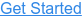Where do you want to go?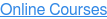# Splitting Trend Lines in QlikView

by Speros Kokenes, on Nov 14, 2013 1:37:43 PM

This post is a follow-up to the Strip Plots post. The Strip Plots post contains more details on the implementation of these charts. See that post for technical background.

Line charts excel at showing trending data. However, when too many lines are plotted at once, the resulting chart can be difficult to interpret:One way to alleviate this is to split the x-axis so that each line has it's own axis with the same domain of values. In the above example, that means that dimension A would have an x-axis from 2010-2013, as would dimension B, etc. All of these lines would share the same y-axis. This can be done in QlikView using a scatter chart: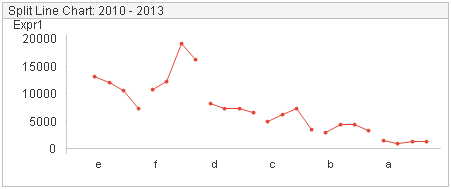In this example, each dimension has a trend line with 4 points plotted. These points correspond with the years 2010-2013. The lines are then organized by highest value at the beginning of the period (2010). This allows the trends of multiple lines to be viewed and compared without cluttering the screen. The chart is created using a scatter chart, where the x-axis is used to assign indices to the points so that they line up appropriate. The x-axis expression looks like this:

=Aggr(NODISTINCT Rank(Aggr(NODISTINCT sum({<Year={\$(=min(Year))}>} Expression1),Dim2)),Dim2) + (Year-min(total Year))/(max(total Year)-min(total Year)+1)

The expression is made up of two parts:

1. Aggr() function to rank each dimension - this aggr function takes an expression and returns an integer ranking. This allows us to sort the order of the lines that will appear by any expression we would like. In this case, the "e" line starts at 1 because it has the highest expression value. The "f" line starts at 2, etc.
2. A function to add spacing to the year to year points on the line - this function checks the year of the data point and places it in the appropriate spot on the axis. Each trend line should be between two integers - for example, "e" extends from 1 - 2. Therefore, we check the current year in relation to the minimum year and divide that by the total range of the period to get a fraction of a distance between 0 and 1. However, to add spacing between each line, we increment the denominator by 1. In practice, this looks like:
 Year Numerator Denominator Total Spacing 2010 (2010-2010)=0 (2013-2010+1)=4 0/4 2011 (2011-2010)=1 4 ¼ 2012 (2012-2010)=2 4 ½ 2013 (2013-2010)=3 4 3/4

Thus, the "e" line is drawn from 1 to 1.75. "f" is drawn from 2 to 2.75, etc.

There are some formatting constraints with this approach that are documented in the Strip Plots post.

Scatter charts can be used in other useful ways to visualize changes over periods like this. For example, directional changes from a start date to an end date can be concisely visualized like so: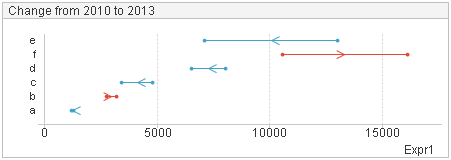This allows the user to view both the magnitude of the dimension value, the magnitude of change, and (thanks to a 0-based axis) the relative percentage change of a dimension. For example, "e" had the largest percentage decrease and appears to be a 50% reduction. "d" and "c" look to have similar size changes, but the change in "c" is more substantial from a percentage perspective. The chart is also color coded to help distinguish the direction of change.

One final example of implementing trend lines with a scatter chart is with indexes. This is useful when a target has been set based on percent changes. For example, imagine a manufacturing company with 6 factories. In 2010, the company sets a goal to reduce emissions across all its factories by 30% in the next 4 years. Using the split trend chart with trend lines indexed by their starting values: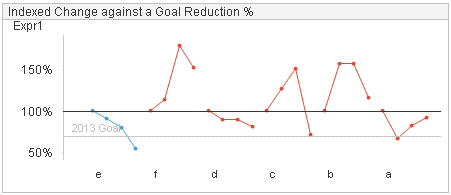Each trend line start at 100%. That is because each line is indexed by the value at the start of the period, 2010. The subsequent trend lines show percentage changes from this starting point. As is indicated by the reference line and the blue color, "e" is the only dimension that reached the target of a 30% reduction.